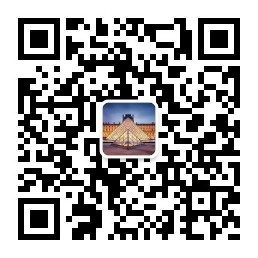# 剑指offer48与49

## 1.面试题48. 最长不含重复字符的子字符串

method1：滑动窗口，使用set去重

``````class Solution {
public:
int lengthOfLongestSubstring(string s) {
// 滑动窗口，维护一个固定窗口

// 存储窗口内的元素
set<char> st;
// 最长字符串的长度
int res = 0;
int l = 0, r = 0;
while(r< s.size() && l <= r) {
// 当前集合中不存在这个元素，右指针后移动
if(st.count(s[r]) == 0) {
st.insert(s[r]);
r++;
res = max(res,r-l);
} else if(st.count(s[r]) > 0) { // 当前集合中存在这个元素，左指针后移动
// 清除l指向的元素
st.erase(s[l]);
l++;
}
}
return res;
}
};``````

method2：滑动窗口，map判重。

``````class Solution {
public:
int lengthOfLongestSubstring(string s) {
// 滑动窗口，维护一个固定窗口

// 存储窗口内的元素
map<char,int> m;
// 最长字符串的长度
int res = 0;
int l = 0, r = 0;
while(r< s.size() && l <= r) {
// 当前集合中不存在这个元素，右指针后移动
if(m[s[r]]==0) {
m[s[r]]++;
r++;
res = max(res,r-l);
} else if(m[s[r]] > 0) { // 当前集合中存在这个元素，左指针后移动
// 清除l指向的元素
m[s[l]]--;
l++;
}
}
return res;
}
};``````

## 2. 面试题49. 丑数

method1：三指针法，初始化为1，三个指针分别指向2，3，5的倍数，每次选择2，3，5中倍数最小的为当前丑数，依次往后2，3，5中谁的倍数最小就将当前的指向后移即可。

``````class Solution {
public:
int nthUglyNumber(int n) {
vector<int> dp(n);
dp = 1;
int p2=0,p3=0,p5=0;
for(int i=1;i<n;i++) {
dp[i] = min(min(2*dp[p2],3*dp[p3]),5*dp[p5]);
if(dp[i] == 2*dp[p2]) p2++;
if(dp[i] == 3*dp[p3]) p3++;
if(dp[i] == 5*dp[p5]) p5++;
}
return dp[n-1];
}
};``````目录